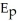# [Solved] Which of the Following Is False Regarding Inelastic Demand

Question 201
Multiple Choice

## Which of the following is FALSE regarding inelastic demand?

A)Price elasticity of demand is less than 1 (< 1).
B)If a firm raises price,total revenues will go up.
C)Price elasticity of demand is greater than 1 (> 1).
D)If a firm lowers price,total revenues will fall.

10+ million students use Quizplus to study and prepare for their homework, quizzes and exams through 20m+ questions in 300k quizzes.

Explore our library and get Economics Homework Help with various study sets and a huge amount of quizzes and questions

3.5K

Study sets

66.5K

Quizzes

5.9M

Questions

Upload material to get free accessInvite a friend and get free accessSubscribe and get an instant access